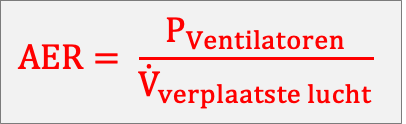BeneluxTo measure the efficiency of air in a data centre, the Airflow Efficiency Ratio (AER) is used. This value reflects the ratio of the power absorbed by the ventilators and the amount of air displaced.

Calculation example

The AER is calculated by dividing the ratio of Pventilators by the displaced air (V) within a given static pressure. The value of the AER is then expressed in Watt (W) / (m³/h).A precision air conditioning unit achieves 40,000 m³/h airflow at a static pressure of 25 Pa. The ventilators have a power consumption of 2.3 kW, or 2,300 Watt.

The AER is then calculated as: AER = 2,300 / 40,000 = 0.057 W/(m³/h).

Applicable for the AER value: the lower the value, the better.